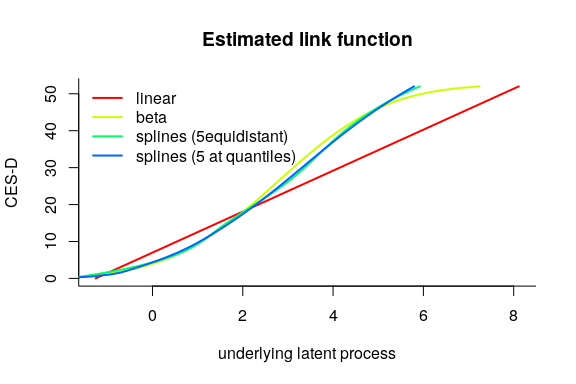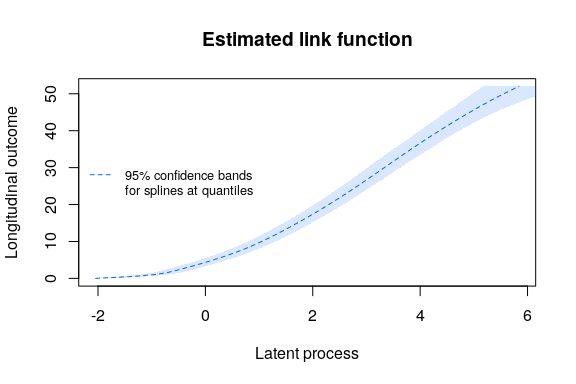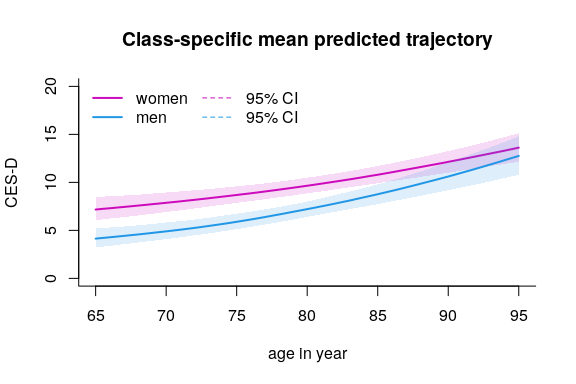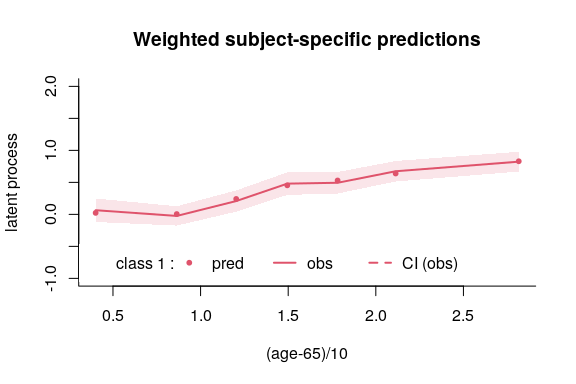# Background on the model

Each dynamic phenomenon can be characterized by a latent process $$(\Lambda(t))$$ which evolves in continuous time $$t$$. When modeling repeated measures of marker, we usually don’t think of it as a latent process measured with error. Yet, this is the underlying assumption made by the mixed model theory. Function lcmm exploits this framework to extend the linear mixed model theory to any type of outcome (ordinal, binary, continuous with any distribution).

## The latent process mixed model

The latent process mixed model is introduced in Proust-Lima et al. (2006 - https://doi.org/10.1111/j.1541-0420.2006.00573.x and 2013 - https://doi.org/10.1111/bmsp.12000 ).

The quantity of interest defined as a latent process is modeled according to time using a linear mixed model:

$\Lambda(t) = X(t) \beta + Z(t)u_i +w_i(t)$

where:

• $$X(t)$$ and $$Z(t)$$ are vectors of covariates ($$Z(t)$$ is included in $$X(t)$$);
• $$\beta$$ are the fixed effects (i.e., population mean effects);
• $$u_i$$ are the random effects (i.e., individual effects); they are distributed according to a zero-mean multivariate normal distribution with covariance matrix $$B$$;
• $$(w_i(t))$$ is a Gaussian process that might be added in the model to relax the intra-subject correlation structure.

The relationship between the latent process of interest and the observations of the marker $$Y_{ij}$$ (for subject $$i$$ and occasion $$j$$) is simultaneously defined in an equation of observation:

$Y_{ij} = H( ~ \Lambda(t_{ij})+\epsilon_{ij} ~ ; \eta)$

where:

• $$t_{ij}$$ is the time of measurement for subject $$i$$ and occasion $$j$$;
• $$\epsilon_{ij}$$ is an independent zero-mean Gaussian error;
• $$H$$ is the link function (parameterized by $$\eta$$) that transforms the latent process into the scale and metric of the marker.

Different parametric families are used. When the marker is continuous, $$H^{-1}$$ is a parametric family of increasing monotonic functions among:

• the linear transformation: this reduces to the linear mixed model (2 parameters)
• the Beta cumulative distribution family rescaled (4 parameters)
• the basis of quadratic I-splines with m knots (m+2 parameters)

When the marker is discrete (binary or ordinal): $$H$$ is a threshold function, that is each level of Y corresponds to an interval of $$\Lambda(t_{ij})+\epsilon_{ij}$$ which boundaries are to be estimated.

## Identifiability

As in any latent variable model, the metric of the latent variable has to be defined. In lcmm, the variance of the errors is 1 and the mean intercept (in $$\beta$$) is 0.

# Example with CES-D

In this vignette, the latent process mixed models implemented in lcmm are illustrated by the study of the linear trajectory of depressive symptoms (as measured by CES-D scale) according to $$age65$$ and adjusted for male. Correlated random effects for the intercept and $$age65$$ are included.

## Model considered:

$CESD_{ij} = H(~ \beta_{1}age65_{ij}+\beta_{2}male_{i}+\beta_{3}age65_{ij}male_{i} +u_{0i}+u_{1i}age65_{ij}+\epsilon_{ij} ~ ; ~ \eta)$

Where : $$u_{i} \sim \mathcal{N}(0,B)$$ and $$\epsilon_{ij} \sim \mathcal{N}(0,1)$$

The Fixed part is $$\beta_{1}age65_{ij}+\beta_{2}male_{i}+\beta_{3}age65_{ij}male_{i}$$ ; the random part is $$u_{0i}+u_{1i}age65_{ij}$$.

## Select the best model

Objects mlin, mbeta, mspl and mspl5q are latent process mixed models that assume the exact same trajectory for the underlying latent process but different link functions: linear,BetaCDF, I-splines with 5 equidistant knots (default with link=‘splines’) and I-splines with 5 knots at percentiles, respectively. To select the most appropriate link function, one can compare these different models. Usually this is achieved by comparing the models in terms of goodness-of-fit using measures such as AIC or UACV.

The summarytable command gives the AIC (the UACV is in the output of each model):

summarytable(mlin,mbeta,mspl,mspl5q,which = c("loglik", "conv", "npm", "AIC"))
loglik conv npm      AIC
mlin   -7056.652    1   8 14129.30
mbeta  -6306.962    1  10 12633.92
mspl   -6509.681    1  13 13045.36
mspl5q -6320.085    1  13 12666.17

In this case, the model with a link function approximated by I-splines with 5 knots placed at the quantiles provides the best fit according to the AIC criterion.

The different estimated link functions can be compared in a plot:

col <- rainbow(5)
plot(mlin, which="linkfunction", bty='l', ylab="CES-D", col=col, lwd=2, xlab="underlying latent process")
legend(x="topleft", legend=c("linear", "beta","splines (5equidistant)","splines (5 at quantiles)"), lty=1, col=col, bty="n", lwd=2)We see that the 2 splines transformations are very close. The linear model does not seem to be appropriate, as shown by the gap betwwen the linear curve and the splines curves. The beta transformation departs from the splines only in the high values of the latent process.

Confidence bands of the transformations can be obtained by the Monte Carlo method :

linkspl5q <- predictlink(mspl5q,ndraws=2000)
legend(x="left", legend=c("95% confidence bands","for splines at quantiles"),lty=c(2,NA), col=c(col,NA), bty="n", lwd=1, cex=0.8)## Postfit outputs

### Summary

The summary of the model includes convergence, goodness of fit criteria and estimated parameters.

summary(mspl5q)
General latent class mixed model
fitted by maximum likelihood method

lcmm(fixed = CESD ~ age65 * male, random = ~age65, subject = "ID",
link = "5-quant-splines", data = paquid)

Statistical Model:
Dataset: paquid
Number of subjects: 500
Number of observations: 2104
Number of observations deleted: 146
Number of latent classes: 1
Number of parameters: 13
0 2 6 12 52

Iteration process:
Convergence criteria satisfied
Number of iterations:  19
Convergence criteria: parameters= 1.3e-08
: likelihood= 1.5e-07
: second derivatives= 3.1e-14

Goodness-of-fit statistics:
maximum log-likelihood: -6320.08
AIC: 12666.17
BIC: 12720.96

Discrete posterior log-likelihood: -6309.09
Discrete AIC: 12644.18

Mean discrete AIC per subject: 12.6442
Mean UACV per subject: 12.6439
Mean discrete LL per subject: -12.6182

Maximum Likelihood Estimates:

Fixed effects in the longitudinal model:

coef      Se    Wald p-value
intercept (not estimated)        0
age65                      0.42421 0.06279   6.756 0.00000
male                      -0.83140 0.19742  -4.211 0.00003
age65:male                 0.23371 0.10300   2.269 0.02327

Variance-covariance matrix of the random-effects:
intercept  age65
intercept   1.89911
age65      -0.39567 0.1711

Residual standard error (not estimated) = 1

coef      Se    Wald p-value
I-splines1 -2.03816 0.13469 -15.132 0.00000
I-splines2  1.04627 0.02461  42.510 0.00000
I-splines3  0.74190 0.03773  19.665 0.00000
I-splines4  0.98399 0.03237  30.400 0.00000
I-splines5  1.55606 0.04480  34.735 0.00000
I-splines6  0.93273 0.16614   5.614 0.00000
I-splines7  1.38790 0.17687   7.847 0.00000

### Graph of predicted trajectories according to a profile of covariates

The predicted trajectories can be computed in the natural scale of the dependent variable and according to a profile of covariates:

datnew <- data.frame(age=seq(65,95,length=100))
datnew$age65 <- (datnew$age - 65)/10
datnew$male <- 0 women <- predictY(mspl5q, newdata=datnew, var.time="age", draws=TRUE) datnew$male <- 1
men <- predictY(mspl5q, newdata=datnew, var.time="age", draws=TRUE)

And then plotted:

plot(women, lwd=c(2,1), type="l", col=6, ylim=c(0,20), xlab="age in year",ylab="CES-D",bty="l", legend=NULL, shades = TRUE)
legend(x="topleft", bty="n", ncol=2, lty=c(1,1,2,2), col=c(6,4,6,4), legend=c("women","men", "95% CI", "95% CI"), lwd=c(2,2,1,1))### Goodness of fit 1: plot of residuals

The subject-specific residuals (qqplot in bottom right panel) should be Gaussian.

plot(mspl5q, cex.main=0.9)

## Goodness of fit 2: plot of predictions versus observations

The mean predictions and observations can be plotted according to time. Note that the predictions and observations are in the scale of the latent process (observations are transformed with the estimated link function):

plot(mspl5q, which="fit", var.time="age65", bty="l", xlab="(age-65)/10", break.times=8, ylab="latent process", lwd=2, marg=FALSE, ylim=c(-1,2), shades=TRUE, col=2)# To go further …

## heterogeneous profiles of trajectories

The latent process mixed model extends to the heterogeneous case with latent classes. The same strategy as explained with hlme (see https://cran.r-project.org/package=lcmm/vignettes/latent_class_model_with_hlme.html ) can be used.

## joint analysis of a time to event

The latent process mixed model extends to the case of a joint model. This is done in Jointlcmm and mpjlcmm.

## multiple markers of the same latent process

In some cases, several markers of the same underlying latent process may be measured. The latent process mixed model extends to that case. This is the purpose of multlcmm (see https://cran.r-project.org/package=lcmm/vignettes/latent_process_model_with_multlcmm.html ).